Kiến thức

# 8.3. Ohm’s law, Joule-Lenz, Wiedemann-Franz, their consideration on the basis of the theory of Drude-Lorentz

By the end of the free run the electron acquires additional kinetic energy, the average value of which is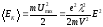. (8.9)

When collided with the ion, electron is assumed to fully transmit acquired additional energy to crystal lattice. This energy is increasing the internal energy of the metal, resulting in its heating. Each electron has in average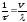collisions 1 s, each time converting energy to the lattice (8.9). Accordingly, in volume unit per time unit heat should be allocated Q: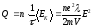,

where n – number of conduction electrons per unit volume, Q – specific heat capacity of current.

According to equation (8.8)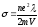, then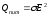.

Or through the current density: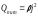, which is Joule-Lenz law.

Let us consider the Wiedemann-Franz law: all metals have a high thermal conductivity. The ratio of the thermal conductivity æ, to the coefficient of conductivity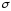is approximately the same for all metals and change in proportion to the absolute temperature. Thus, at room temperature it is 5,8∙10-6 J∙Ω/s∙C for aluminum, 6,4∙10-6 J∙Ω/s∙C for copper, 7,0∙10-6 J∙Ω/s∙C for lead.

Non-crystalline metals aslo have the ability to conduct heat, but the thermal conductivity of metals is much higher than the thermal conductivity of dielectrics. Then we can conclude that the heat is transfered not by crystal lattice, but by free electrons. Considering these electrons as a monatomic gas, thermal conductivity can be used for expression of the kinetic theory of gases:

æ=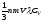.

Lecture 9

Chapter 9 The contact and volume differences of potential

9.1 The work of electrons relasing

The conduction electrons, which are due to classical theory of electric conductivity create the so-called “electron gas”, take part in the thermal motion. As the result of the conduction electrons are held inside the metal, we claim that near the surface of the metal they are influenced by the forces directed to the inside of metal. The electron can leave the surface of the metal only when it can do the work against these forces. This work is called inside work function.

If the electron left the surface of the metal, then it would have the positive charge on its surface and due to the Coulomb’s law it interacts with gone electron and turns it back.

The work against this force is a part of work function. The fastest electrons in the thermal motion can move away from the surface of the metal on several intermolecular distances. In this way, the cloud which is formed by the electrons. The density of the cloud decreases rapidly with the distance from the surface, which becomes positively charged.

Positively charged metal surface and negatively charged cloud forms “capacitor” (two arms, one of which is positively charged, while the other – negative). The field will be centered between the “shell”. It will be no electrical field outside of it. In order to pass this double layer, the electron should do the work, that is the absolute work function caused by two abovementione conditions.

A potential difference Δφ. Between the surface and cloud is this potential difference is called potential barrier. It opposes the rise of the relasing process, as the certain work function has to be done to overcome the potential barrier at the releasing of electrons from the metal.

If the electron inside of the metal has kinetic energy Ek, it can leave the metal surface, provided that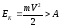.

The work function is difined by: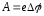.

The work function is expressed in electron volts (eV). 1 eV – the energy acquired by an electron, which has passed the potential difference of 1V. 1 eV = 1V ∙ 1,6 ∙ 10-19Kl = 1,6 ∙ 10-19Dzh.

At room temperature, the number of electrons, with speed sufficient for electron escape of the metal is very small, since the work function of the metal has of the order of several electron volts, and the kinetic energy of the electron is determined by the energy of its thermal motion at this temperature (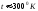) :

kT = 1.38 ∙ 10-23 (J / K) ∙ 300 (K) = 4,15 ∙ 10-29Dzh = 0.026 eV.

Electron work function of copper is 4.2 eV tungsten – 4.5 eV with lithium – 2.3 eV, platinum – 6.3 eV.

9.2 Thermionic emission.

Thermionic emission is a phenomenon of the release of electrons from a substance under the influence of temperature which exists due to thermal excitation energy. The mechanism of thermionic emission: for every electron that leaves the metal surface it is possible to illustrate the potential energy dependence on thermionic emission (9.2).

The electron inside the metal is not affected by any force , thus potential energy does not change.

To make a full diagram of potential energy for the metal it worth mentioning that:

-Vacuum-metal boundary is a potential barrier;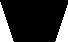W

0 x

Wn

Wf

fig.9.1

– the metal in general for electron is presented as a potential well with the depth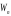;

– the allowed values of potential energy of the electron inside the metal is presented as very closely located discrete levels.

At temperature T = 0 K, electrons fill the lowest level in the potential well to some top level. This level is called the Fermi level and the energy that corresponds to the highest filled levels – Fermi energy (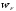). Thus at T = 0 K electron energy is in the range: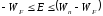.

It is obvious that under such conditions the electron is not able to leave the metal , but if electron is given additional energy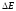( for example, light irradiation), than it can break the border.

This additional energy should be not less than difference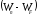, that is minimum energy which is needed to release the electron from metal is called work function :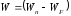.

At T> 0 K movement of electrons are more active of the expense of the energy of thermal excitation, the electrons are redistributed to the public. In some cases, electrons receive from the lattice extra energy which is greater than the work of thear release and they have the ability to leave the metal. This phenomenon is called thermionic emision. Fermi energy is the energy which electron has at the temperature 0 K.

The phenomenon of thermionic emission is used in the diods . If there is a field in the vacuum electronic environment of the heated metal which is directed from the metal surface , it will copture electrons and current flows through the vacuum. This current is called thermionic.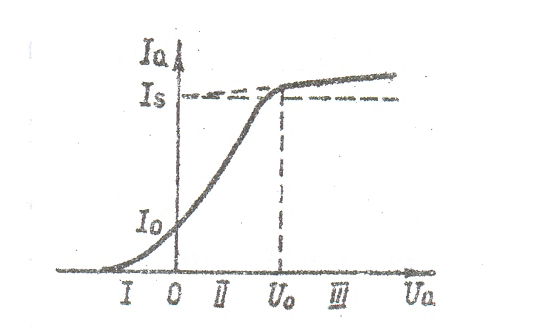fig.9.2

9.3 Thermionic phenomenon. The thermo-emf. The Seebeck effect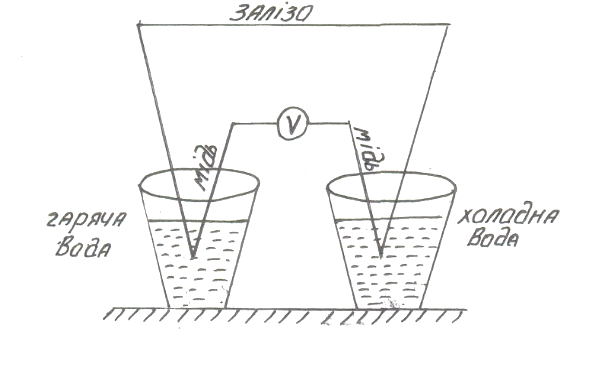fig.9.3

The idea of Seebeck effect is in the closed electric circle which consists of two different metals the thermoelectric power is appeared when jointes have different temperature.

Two metals are called thermocouple and current is called thermoelectric.

Thermoelectric power (thermopower) exists in two types: contact and volume. Consider two cases.

1. Two conductors of different kinds brought into contact. In point of contact electron equilibrium is disturbed due to the potential difference in conductors.

For part of the electrons it is energetically favorable to move from one conductor to another. They stay in close contact to the second conductor under the affected charge which is not compensated by the second conductor.

At the contact the double electronic layer is formed, which can be viewed as a flat capacitor of atomic thickness. The process continues until the electric field of the double layer is increased to such a value that will prevent further transfer of electrons.

The voltage drop in this double layer is called the contact potential difference. The contact potential difference depends on the temperature of contact T.Ek(T1) Ek(T2)

—– —–

+++ +++

fig.9.3.

There are two thermocouples which contact thermopower in the hot and cold junctions. When traversing range of the thermocouple clockwise, full growth potential at junctions is numerically equal to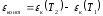.

This value is called the contact component of thermopower. It is caused by the temperature dependence of the contact potential difference.

1. If the conductor is heated un evenly along the length, the balance of electrons

in the volume is broken and the electrons flow from one area conductor to the other. There is a distribution of charges in the conductor that create an electric field. This process continues until the field will not be an obsticle to subsequent redistribution of electrons.

The field intensity E will be proportional to the gradient of the temperature of the conductor: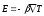,

where β – coefficient of proportionality, which depends on the type of conductor. If we integrate the expression for the field intensity along the length, we obtain a potential difference.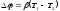.

In case of thermocouples there are two potential differences: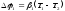,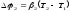.

Accordingly, the traversing range of the thermocouple clockwise increase the volumetric component of potential: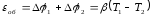,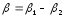.

εob – spatial component of thermopower. Typically, the hot end of the wire and the average energyα1

++++ ——-

++++ ——-

α2

fig.9.4

density of conduction electrons is higher than the cold end. Therefore, current will flow from the hot end to the cold, as a result, there is a lack of electrons on the cold end and surplus on the hot one.

Full thermopower – is the algebraic sum of the contact and bulk component thermopower, while traversing a clockwise circle thermocouple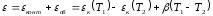.

Lecture 10

Section 7.Electric current in liquids

Chuyên mục: Kiến thức